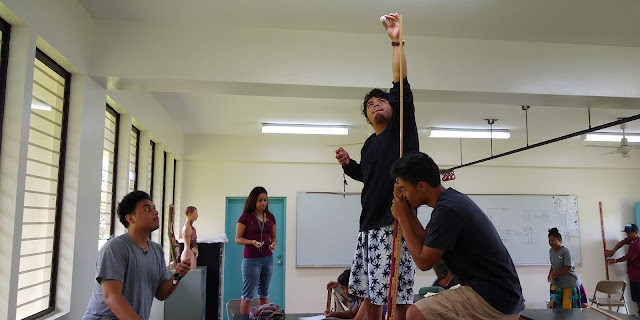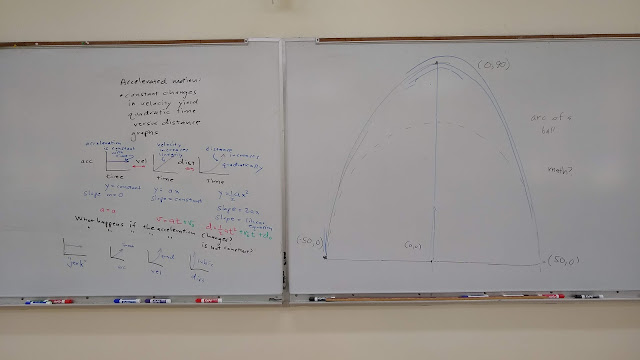### Five days of acceleration

The second problem on the Friday quiz at the end of week two provided the segue to the acceleration curve I intended to generate on Monday of the third week. The goal of that third week Monday acceleration exercise was to generate a curve that will be seen again in the Thursday laboratory.

The Monday run began at the zero meter distance mark at the post I am holding. To the best of my ability I began with a zero meter per second speed. Chalk marks were at one, two three, five, seven, and nine meters. Four people had chronographs which proved prescient as in an given run only two of the four timers felt that they had usable data.

Although I attempted one practice and two trial runs with my ASIC GT 2000 6 trail shoes, the soles lived up to the slipperiness I experienced in them while on Kosrae this past summer. Once wet these shoes have all of the traction of zoris on a wet tile floor, in other words, no traction. On the second trial my feet slid off of the board, and I wasn't even driving the RipStik that hard.

So I pulled my shoes off and did what any five year old does on RipStik around here: rides barefoot for better control. Being barefoot gave me the grip I needed to drive the board. Two trials later, however, and the data did not appear to be very consistent with a steady increase in velocity. A time check showed 12:38 and the new end time for class is 12:50. With limited time, I went with data taken by Rangpino - his data appeared to be the most consistent.

I recorded the data on a sheet of paper, and then ran a graph in Desmos.

In class I entered only the first table. I was surprised that the data appeared to be a relatively smooth curve, I had not seen that in the raw data review when I had rolled through the memory of the stopwatch. I then ran the regression d₁~½at² I was even more than surprised when the regression ran through the data points with a correlation of 0.9993. As usual, I assigned the data as an assignment to be completed using Desmos.

Once class was over and I returned to the office I put the data into a spreadsheet. As seen in the Desmos chart above, the rate of increase in the velocity was fairly constant; linear increase in velocity being a necessary condition to produce a parabolic time versus distance graph.

 time (s) distance (m) time (s) velocity (m/s) acc (m/s²) 0 0 0.00 0.00 0.37 2.12 1 2.12 0.47 0.22 3.41 2 3.41 0.78 0.24 4.16 3 4.16 1.33 0.74 5.38 5 5.38 1.64 0.25 6.47 7 6.47 1.83 0.18 7.32 9 7.32 2.35 0.61

An analysis of the velocity data produced a best fit slope of 0.30 m/s² (with the y-intercept forced to be zero) against the predicted slope of 0.338 m/s². Note that the slope of the velocity versus time line is the acceleration. 0.338 m/s² is the best fit acceleration from the parabolic data. In this system, the difference between the acceleration and the best fit slope of the velocity reflects the degree to which the velocity data is not perfectly linear. Ideally these would be the same.

An analysis of the acceleration shows that the acceleration was not constant, but the differences from a constant acceleration were, for the most part, small, and due both to real changes in acceleration and timing errors. Ideally the acceleration data would generate a line with a slope of zero and a y-intercept equal to the constant acceleration. The best fit line for the acceleration data is the line y = 0.0227 + 0.305.

Note that in the Desmos chart above I used the acceleration a₁ from the quadratic regression to generate the line for the velocity data and the constant line for the acceleration data. The points plotted come from the spreadsheet calculations.

Wednesday I switched back to the North Face shoes that give me solid traction on the RipStik. I began the class by going over the data from Monday.

Then I laid down three graphs in sequence, starting with a constant acceleration versus time as a horizontal line, generating a constantly rising velocity on a second graph, generating a parabola on a time versus distance graph. I integrated, while explaining that I was doing so. I also explained the variables in the equations.

I went on to cover the slope of a quadratic equation. I noted that my argument was a hand waving argument, that I was running only on the data fitting.

I then showed on the laptop/television combination that the velocity was rising fairly constantly, and the acceleration was roughly flat. I used Desmos' capability to not show points and lines and had kept these hidden until I wanted them to appear.

Then I explained that I wanted the class to see a full parabola, and we returned to the RipStik test track to use the slope. I tried a ball over lunch, but it would not roll back down without winding up in the gutter.

Timing marks were at 0, 3, 6, 8, 9 and apex which was marked by Michsane.

The data came out better than in the past two terms which had seen distinctly inverted vee shapes.

Only the vertex was problematics, otherwise the data was fairly quadratic. This term I remembered to drive harder downhill, to not just coast, but to intentionally accelerate downhill. The uphill decelerates naturally. There is too much friction to let the slope generate the downward acceleration.

And the acceleration comes out negative - a negative lead coefficient leads to an inverted parabola.

On Thursday the laboratory explored the nature of falling objects via dropped balls. I opened the class a little more carefully and mathematically. I did not make clear that we would be measuring the acceleration of gravity. I started by noting that a ball held above the ground has a velocity of zero, and once released has a velocity. Thus there must be, if nothing else, at least an initial acceleration. I then asked what happens with the speed after the release: does the speed remain the same (referencing last week and constant velocity objects) or does the speed increase (referencing Monday's RipStik run). And if the velocity increases, does the rate of increase remain constant? These are subtle ideas to wrap one's mind around.

The above board captures the approach this term. Does the ball fall at a constant speed (a line on a time versus distance graph) or does the ball fall faster and faster (a curve on a time versus distance graph)?

What is the nature of the relationship between time and distance for a falling ball? And if the velocity is increasing, is that rate of increase a steady rate of increase? If the rate of increase in the velocity is constant - then a parabola is produced on a time versus distance graph, a line with a slope equal to the acceleration is produced on a velocity versus time graph, and a horizontal line is produced on an acceleration versus time graph.

I then outlined the procedure that would be used, noting that the distances would be measured again in meters. Thus far meters have worked well, perhaps better, than centimeters.

Rangpino and Bredalyn

Drops from one and two meters were done inside.

Franky, Skyler, and Dori-An

As was done last spring, no drops were performed from three meters as a safety precaution.Keanu, Luke, Shawn

That said, one group opted to chase three meter drops in the afternoon class.Drops from four and five meters were done again from the porch.Only upstairs in A204, in the second half of the laboratory, after graphing data, did I reveal the existence of a known acceleration of gravity.Small changes can make big differences. On Friday I wanted to review, reinforce, tie together again the relationship of acceleration, velocity, and distance.So I began with the material on the left board prior to launching into the "find the equation" exercise based on a ball arc on the board. This actually sidefired: I had inadvertently put an equation on the board that could be used in Desmos to reverse engineer the equation. The dynamics of any two classes differs. Nearly every group started to head down the path of putting the data into Desmos and then running an equation against the arc using Desmos. This meant that essentially all of the groups had stumbled onto a viable equation in roughly 12 minutes. That was different than last spring, and perhaps the board introduction set that up in part. Maybe this exercise has to be run off of a cold start, blank board, to obtain the effect I obtained last spring. This is the mystery of teaching - the same basic exercise run twice will behave differently. As magicians and comics are keenly aware, the set-up is everything to the success of the show. Here too perhaps the board changed the conditions, perhaps just the mix of students. This is the class where nearly every student has a capable smartphone. There is also a depth of capacity inside the class, including a number of students who have worked in less structured assignment environments.

On Monday at the start of the fourth week I had the students put their equations on the board. All of the groups put up the same equation, thus I did not have the groups present their results. I simply noted that all of the groups had arrived at the same math and that this was a core idea in the course. Underneath any system are equations and that observers of the system will agree on the equation.

The second part of the class on Monday addressed a common misconception echoed in the laboratory three laboratory reports: that the student had measured the speed. On Monday I noted that they had not measured the speed on the prior Thursday. On the contrary, they had measured the time to fall a particular distance. And that while this could be used to calculate a speed, that would be an average speed for the ball over that distance, not the speed of the ball at the bottom of that distance. Some students had averred that they were measuring the speed with which the ball hit the floor. I noted that the parabola guaranteed, however, that the speed was steadily increasing. This was the fact that the parabola supported: an ever increasing speed. Thus as the ball fell from one meter up, the speed was ever increasing. The distance divided by the time provided only an average speed, which occurred at some point on the way down.

This provided a natural segue into "What is the speed at the bottom?" Noting that I had no way to measure a vertical speed, I suggested that I could run a series of measurements of height versus speed using the RipStik, provided I measured only the vertical drop and not the distance along the slope.

I had already remeasured the heights prior to class. Taking the class outside I gathered height versus bottom speed data for the RipStik. This segue provides a chance to tell a story with logical transitions. We explored first how velocity relates to slope, chasing after the meaning of curvature in the above data gathering exercises. This story then continues into kinetic energy by the next Wednesday. This term a modification to the fourth week laboratory and the following Friday may provide the next link in the chain to introduce force from a momentum perspective, providing another logical step in the story that weaves through the motion section of the course.